# Anomaly Detection using Deep Learning¶

## Demo¶

We'll train a neural network called an Autoencoder on a credit card fraud dataset from Kaggle without labels. It will learn how to reconstruct the input data after time. Then, we'll use its learned representations to turn to perform binary classification.

## What's an autoencoder?¶Auto-encoder is a type of neural network that approximates the function:

f(x) = x.

Basically, given an input x, network will learn to output f(x) that is as close as to x.

The error between output and x is commonly measured using root mean square error (RMSE)

mean((f(x) – x) ^ 2)

which is the loss function we try to minimise in our network.

Autoencoders follows a typical feed-forward neural networks architecture except that the output layer has exactly same number of neurons as input layer. And it uses the input data itself as its target. Therefore it works in a way of unsupervised learning – learn without predicting an actual label

The ‘encoder’ – job is to ’embed’ the input data into a lower dimensional array. The ‘decoder’ - job is to try to decode the embedding array into the original one.

We can have either one hidden layer, or in the case below, have multiple layers depending on the complexity of our features.

## What are other Anomaly Detection methods?¶

PCA - Principal Component analysis. This is actually a dimensionality reduction technique but can be used to detect anomalies since when visualized, the learned 'principal components' will show outliers.K means - This is simple arithmetic, a very popular algorithm. Gives great results for small datasets, utility declines as your data grows >1000 data points.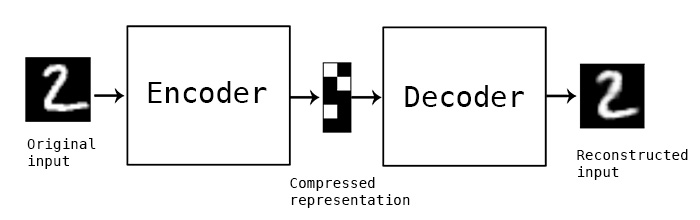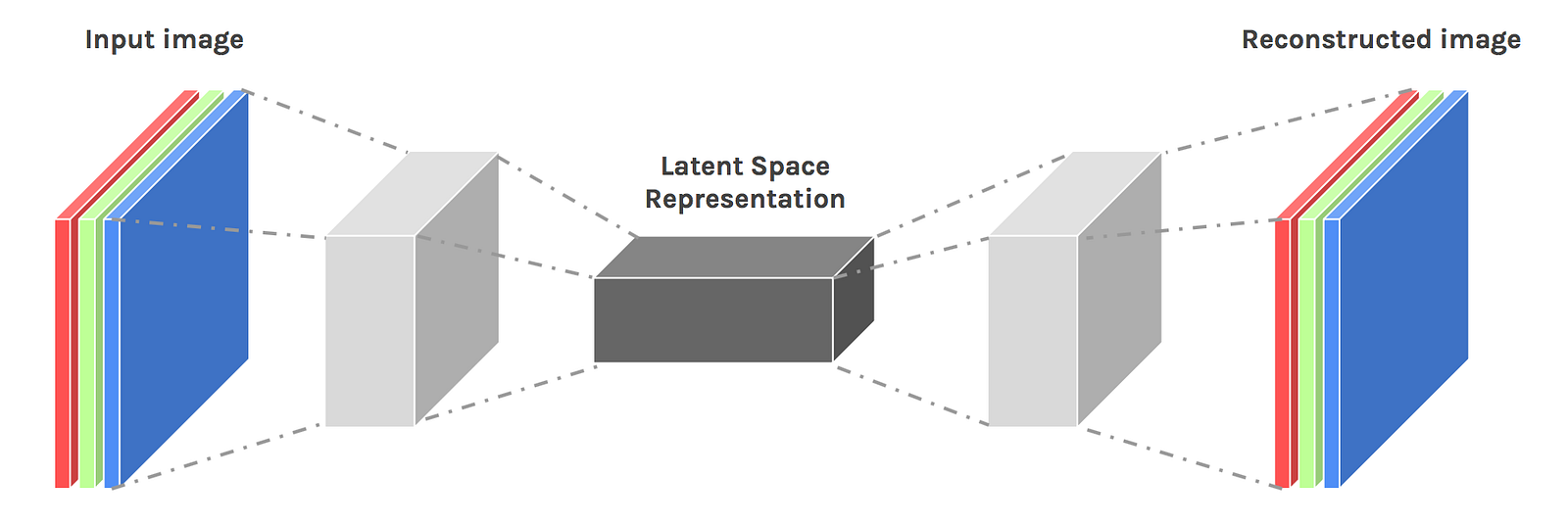## What are the steps¶

1. First part of the forward pass - encode the data points into an encoded representation
2. Second part of the forward pass - decode the data points
3. Measure the Root mean squared error. Minimize it using backpropagation.
4. We'll define a threshold value. If the RMSE is above that threshold value then, it's considered an anomaly. We can Rank the RSME values and the top .1 can be considered anomalies.

The reason the top X datapoints with the highest RMSE error are considered anomalies is because the RMSE will measure the difference between the learned patterns and the anomaly value. Since the majority of the datapoints share similar patterns, the anomalies will stick out. They'll have the highest difference.

So we're basically creating a generalized latent representation of all the datapoints (both fraud and valid) and since the valid datapoints far outweight the fraud datapoints, this learned representation will be one for valid datapoints. Then when the difference between the learning and the fraud datapoint is measured, it will be very high. Anomaly spotted!

## How can this be applied at CERN?¶

Why use deep learning? -Particle data is very high dimensional -It works well on big datasets and big computing power -No need to perform feature engineering, deep nets will learn the most relevant features.

-Classification for known particles -Generative models for simulations (Generative adversarial networks, variational autoencoders) -Clustering (What we're talking about)In :
import pandas as pd #data manipulation
import numpy as np #matrix math
import tensorflow as tf #machine learning
import os #saving files
from datetime import datetime #logging
from sklearn.metrics import roc_auc_score as auc  #measuring accuracy
import seaborn as sns #plotting

In :
import matplotlib.pyplot as plt #plotting
import matplotlib.gridspec as gridspec
%matplotlib inline


## Data Exploration¶

In :
data_dir = "C:\\Users\\weimin\\Desktop\\Fraud"

In :
df.shape

Out:
(284807, 31)
In :
print("Total time spanning: {:.1f} days".format(df['Time'].max() / (3600 * 24.0)))
print("{:.3f} % of all transactions are fraud. ".format(np.sum(df['Class']) / df.shape * 100))

Total time spanning: 2.0 days
0.173 % of all transactions are fraud.

In :
df.head()

Out:
Time V1 V2 V3 V4 V5 V6 V7 V8 V9 ... V21 V22 V23 V24 V25 V26 V27 V28 Amount Class
0 0.0 -1.359807 -0.072781 2.536347 1.378155 -0.338321 0.462388 0.239599 0.098698 0.363787 ... -0.018307 0.277838 -0.110474 0.066928 0.128539 -0.189115 0.133558 -0.021053 149.62 0
1 0.0 1.191857 0.266151 0.166480 0.448154 0.060018 -0.082361 -0.078803 0.085102 -0.255425 ... -0.225775 -0.638672 0.101288 -0.339846 0.167170 0.125895 -0.008983 0.014724 2.69 0
2 1.0 -1.358354 -1.340163 1.773209 0.379780 -0.503198 1.800499 0.791461 0.247676 -1.514654 ... 0.247998 0.771679 0.909412 -0.689281 -0.327642 -0.139097 -0.055353 -0.059752 378.66 0
3 1.0 -0.966272 -0.185226 1.792993 -0.863291 -0.010309 1.247203 0.237609 0.377436 -1.387024 ... -0.108300 0.005274 -0.190321 -1.175575 0.647376 -0.221929 0.062723 0.061458 123.50 0
4 2.0 -1.158233 0.877737 1.548718 0.403034 -0.407193 0.095921 0.592941 -0.270533 0.817739 ... -0.009431 0.798278 -0.137458 0.141267 -0.206010 0.502292 0.219422 0.215153 69.99 0

5 rows × 31 columns

In :
df.columns

Out:
Index(['Time', 'V1', 'V2', 'V3', 'V4', 'V5', 'V6', 'V7', 'V8', 'V9', 'V10',
'V11', 'V12', 'V13', 'V14', 'V15', 'V16', 'V17', 'V18', 'V19', 'V20',
'V21', 'V22', 'V23', 'V24', 'V25', 'V26', 'V27', 'V28', 'Amount',
'Class'],
dtype='object')
In :
df.dtypes

Out:
Time      float64
V1        float64
V2        float64
V3        float64
V4        float64
V5        float64
V6        float64
V7        float64
V8        float64
V9        float64
V10       float64
V11       float64
V12       float64
V13       float64
V14       float64
V15       float64
V16       float64
V17       float64
V18       float64
V19       float64
V20       float64
V21       float64
V22       float64
V23       float64
V24       float64
V25       float64
V26       float64
V27       float64
V28       float64
Amount    float64
Class       int64
dtype: object
In :
plt.figure(figsize=(12,5*4))
gs = gridspec.GridSpec(5, 1)
for i, cn in enumerate(df.columns[:5]):
ax = plt.subplot(gs[i])
sns.distplot(df[cn][df.Class == 1], bins=50)
sns.distplot(df[cn][df.Class == 0], bins=50)
ax.set_xlabel('')
ax.set_title('histogram of feature: ' + str(cn))
plt.show()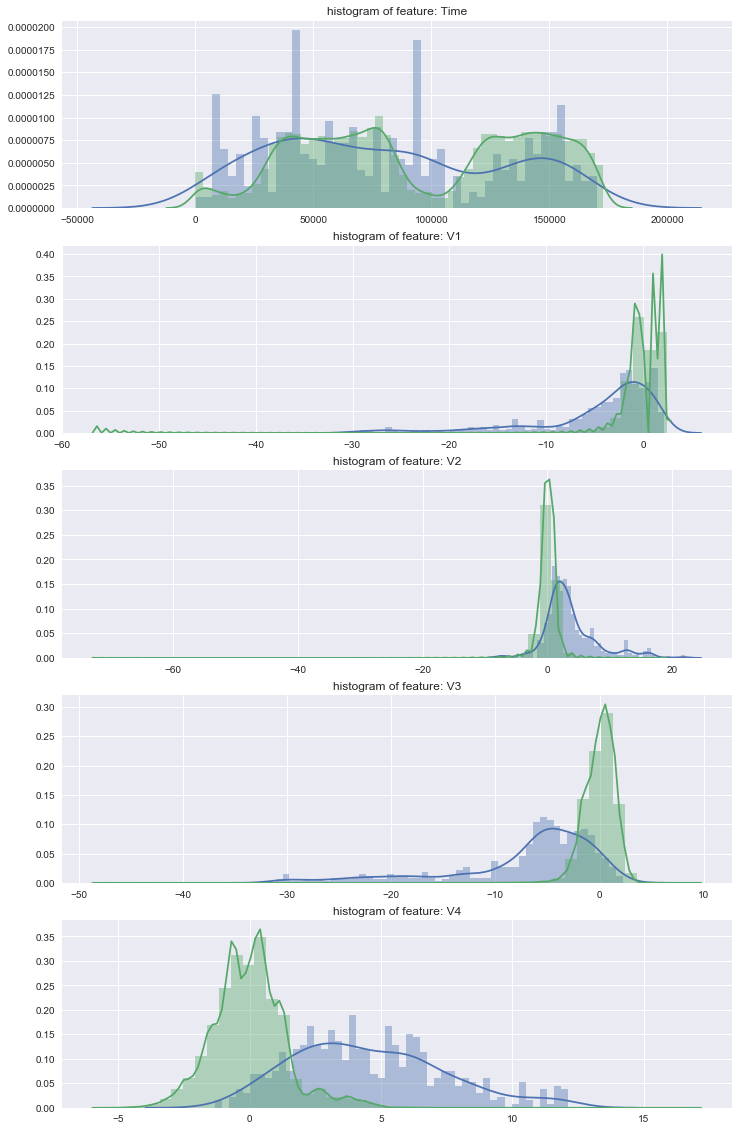## Train and test split on time series, using first 75% as training/val, and last 25% as test¶

In :
TEST_RATIO = 0.25
df.sort_values('Time', inplace = True)
TRA_INDEX = int((1-TEST_RATIO) * df.shape)
train_x = df.iloc[:TRA_INDEX, 1:-2].values
train_y = df.iloc[:TRA_INDEX, -1].values

test_x = df.iloc[TRA_INDEX:, 1:-2].values
test_y = df.iloc[TRA_INDEX:, -1].values

In :
print("Total train examples: {}, total fraud cases: {}, equal to {:.5f} of total cases. ".format(train_x.shape, np.sum(train_y), np.sum(train_y)/train_x.shape))

Total train examples: 213605, total fraud cases: 398, equal to 0.00186 of total cases.

In :
print("Total test examples: {}, total fraud cases: {}, equal to {:.5f} of total cases. ".format(test_x.shape, np.sum(test_y), np.sum(test_y)/test_y.shape))

Total test examples: 71202, total fraud cases: 94, equal to 0.00132 of total cases.


2 types of standardization z-score and min-max scaling.

z-score will normalize each column into having mean of zero and standardization of ones, which will be good choice if we are using some sort of output functions like tanh, that outputs values on both sides of zero. Besides, this will leave values that are too extreme to still keep some extremeness left after normalization (e.g. to have more than 2 standard deviations away). This might be useful to detect outliers in this case.

The second min-max approach will ensure all values to be within 0 ~ 1. All positive. This is the default approach if we are using sigmoid as our output activation.

Just to recap the differences between sigmoid and tanh below (sigmoid will squash the values into range between (0, 1); whereas tanh, or hyperbolic tangent, squash them into (-1, 1)):Based on experiments, I found tanh to perform better than sigmoid, when using together with z-score normalization. Therefore, I chose tanh followed by z-score.

## Feature Normalization - min max score (used for sigmoid activation)¶

In :
'''cols_max = []
cols_min = []
for c in range(train_x.shape):
cols_max.append(train_x[:,c].max())
cols_min.append(train_x[:,c].min())
train_x[:, c] = (train_x[:, c] - cols_min[-1]) / (cols_max[-1] - cols_min[-1])
test_x[:, c] =  (test_x[:, c] - cols_min[-1]) / (cols_max[-1] - cols_min[-1])'''


## Feature Normalization 2 - z score (for tanh activation)¶

In :
cols_mean = []
cols_std = []
for c in range(train_x.shape):
cols_mean.append(train_x[:,c].mean())
cols_std.append(train_x[:,c].std())
train_x[:, c] = (train_x[:, c] - cols_mean[-1]) / cols_std[-1]
test_x[:, c] =  (test_x[:, c] - cols_mean[-1]) / cols_std[-1]


## 1. Auto-encoder as unsupervised learning¶

### Parameters¶

In :
# Parameters
learning_rate = 0.01 #how fast to learn? too low, too long to converge, too high overshoot minimum
training_epochs = 10
batch_size = 256
display_step = 1

# Network Parameters (neurons )
n_hidden_1 = 15 # 1st layer num features
#n_hidden_2 = 15 # 2nd layer num features
n_input = train_x.shape # MNIST data input (img shape: 28*28)


### Train and val the model - (1 hidden layer turned out to be enough)¶

In :
X = tf.placeholder("float", [None, n_input])

weights = {
'encoder_h1': tf.Variable(tf.random_normal([n_input, n_hidden_1])),
#'encoder_h2': tf.Variable(tf.random_normal([n_hidden_1, n_hidden_2])),
'decoder_h1': tf.Variable(tf.random_normal([n_hidden_1, n_input])),
#'decoder_h2': tf.Variable(tf.random_normal([n_hidden_1, n_input])),
}
biases = {
'encoder_b1': tf.Variable(tf.random_normal([n_hidden_1])),
#'encoder_b2': tf.Variable(tf.random_normal([n_hidden_2])),
'decoder_b1': tf.Variable(tf.random_normal([n_input])),
#'decoder_b2': tf.Variable(tf.random_normal([n_input])),
}

# Building the encoder
def encoder(x):
#input times weight + bias. Activate! It rhymes.
# Encoder Hidden layer with sigmoid activation #1
biases['encoder_b1']))
return layer_1

# Building the decoder
def decoder(x):
# Encoder Hidden layer with sigmoid activation #1
biases['decoder_b1']))
return layer_1

# Construct model
#feed the input data into the encoder.
encoder_op = encoder(X)
#and learned representation to the decoder
decoder_op = decoder(encoder_op)

# Prediction
y_pred = decoder_op
# Targets (Labels) are the input data.
y_true = X

# Define batch mse
batch_mse = tf.reduce_mean(tf.pow(y_true - y_pred, 2), 1)

# Define loss and optimizer, minimize the squared error
cost = tf.reduce_mean(tf.pow(y_true - y_pred, 2))
optimizer = tf.train.RMSPropOptimizer(learning_rate).minimize(cost)

# TRAIN StARTS
save_model = os.path.join(data_dir, 'temp_saved_model_1layer.ckpt')
saver = tf.train.Saver()

# Initializing the variables
init = tf.global_variables_initializer()

with tf.Session() as sess:
now = datetime.now()
sess.run(init)
total_batch = int(train_x.shape/batch_size)
# Training cycle
for epoch in range(training_epochs):
# Loop over all batches
for i in range(total_batch):
#pick a random datapoint from the batch
batch_idx = np.random.choice(train_x.shape, batch_size)
batch_xs = train_x[batch_idx]
# Run optimization op (backprop) and cost op (to get loss value)
#recursive weight updating via gradient computation.
_, c = sess.run([optimizer, cost], feed_dict={X: batch_xs})

# Display logs per epoch step
if epoch % display_step == 0:
train_batch_mse = sess.run(batch_mse, feed_dict={X: train_x})
print("Epoch:", '%04d' % (epoch+1),
"cost=", "{:.9f}".format(c),
"Train auc=", "{:.6f}".format(auc(train_y, train_batch_mse)),
"Time elapsed=", "{}".format(datetime.now() - now))

print("Optimization Finished!")

save_path = saver.save(sess, save_model)
print("Model saved in file: %s" % save_path)


Epoch: 0001 cost= 0.411727011 Train auc= 0.956021 Time elapsed= 0:00:01.806808
Epoch: 0002 cost= 0.846064389 Train auc= 0.953359 Time elapsed= 0:00:03.255659
Epoch: 0003 cost= 0.354484618 Train auc= 0.953008 Time elapsed= 0:00:04.644354
Epoch: 0004 cost= 0.521521986 Train auc= 0.953450 Time elapsed= 0:00:06.098230
Epoch: 0005 cost= 0.517319083 Train auc= 0.953781 Time elapsed= 0:00:07.534039
Epoch: 0006 cost= 0.679800570 Train auc= 0.954154 Time elapsed= 0:00:08.928748
Epoch: 0007 cost= 0.325362355 Train auc= 0.953365 Time elapsed= 0:00:10.329510
Epoch: 0008 cost= 0.411871493 Train auc= 0.953527 Time elapsed= 0:00:11.756268
Epoch: 0009 cost= 0.280223519 Train auc= 0.954088 Time elapsed= 0:00:13.173037
Epoch: 0010 cost= 1.056191683 Train auc= 0.953535 Time elapsed= 0:00:14.504578
Optimization Finished!
Model saved in file: C:\Users\weimin\Desktop\Fraud\temp_saved_model_1layer.ckpt


### Test model - on later 25% test data¶

In :
save_model = os.path.join(data_dir, 'temp_saved_model_1layer.ckpt')
saver = tf.train.Saver()

# Initializing the variables
init = tf.global_variables_initializer()

with tf.Session() as sess:
now = datetime.now()

saver.restore(sess, save_model)

test_batch_mse = sess.run(batch_mse, feed_dict={X: test_x})

print("Test auc score: {:.6f}".format(auc(test_y, test_batch_mse)))


INFO:tensorflow:Restoring parameters from C:\Users\weimin\Desktop\Fraud\temp_saved_model_1layer.ckpt
Test auc score: 0.943175


## Visualize the prediction¶

### 1. Show distribution of all mse (fraud scores if you want to call it)¶

In :
plt.hist(test_batch_mse, bins = 100)
plt.show()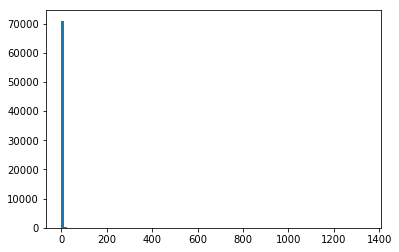### Zoom into (0, 20) range¶

In :
plt.hist(test_batch_mse[test_batch_mse < 20], bins = 100)
plt.show()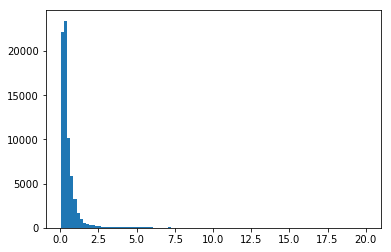### 2. Display only fraud cases¶

In :
plt.hist(test_batch_mse[test_y == 1.0], bins = 100)
plt.show()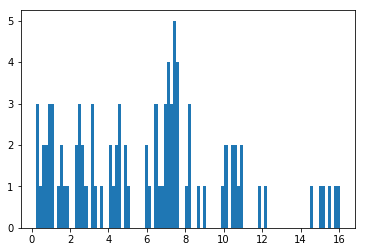In :
THRE_TEST = 7
print(" All scores above treshold: {}, all pos above threshold: {}, and percentage of accuracy above treshold are: {}".format( \
np.sum(test_y[test_batch_mse > THRE_TEST]), np.sum(test_batch_mse > THRE_TEST), np.sum(test_y[test_batch_mse > THRE_TEST]) / np.sum(test_batch_mse > THRE_TEST)))


 All scores above treshold: 41, all pos above threshold: 561, and percentage of accuracy above treshold are: 0.07308377896613191


## 2. Build a binary classifier model that output probabilities of being fraud, using previous auto-encoder embedding layer as model input for all data¶

### 1) Get auto-encoder embedding (encoder_op) for both train and test data¶

In :
save_model = os.path.join(data_dir, 'temp_saved_model_1layer.ckpt')
saver = tf.train.Saver()

# Initializing the variables
init = tf.global_variables_initializer()

with tf.Session() as sess:
now = datetime.now()
saver.restore(sess, save_model)

test_encoding = sess.run(encoder_op, feed_dict={X: test_x})
train_encoding = sess.run(encoder_op, feed_dict={X: train_x})

print("Dim for test_encoding and train_encoding are: \n", test_encoding.shape, '\n', train_encoding.shape)

INFO:tensorflow:Restoring parameters from C:\Users\weimin\Desktop\Fraud\temp_saved_model_1layer.ckpt
Dim for test_encoding and train_encoding are:
(71202, 15)
(213605, 15)


### 2) Build the graph for FC layers (best hidden size based on validation is found to be 4)¶

In :
#n_input = test_encoding.shape
n_input = test_encoding.shape

hidden_size = 4
output_size = 2

X = tf.placeholder(tf.float32, [None, n_input], name='input_x')
y_ = tf.placeholder(tf.int32, shape=[None, output_size], name='target_y')

weights = {
'W1': tf.Variable(tf.truncated_normal([n_input, hidden_size])),
'W2': tf.Variable(tf.truncated_normal([hidden_size, output_size])),
}
biases = {
'b1': tf.Variable(tf.zeros([hidden_size])),
'b2': tf.Variable(tf.zeros([output_size])),
}

pred_probs = tf.nn.softmax(pred_logits)

cross_entropy = tf.reduce_mean(
tf.nn.softmax_cross_entropy_with_logits(labels=y_, logits=pred_logits))



### 3) Prepare the data set. Now we need to re-split the train into train/val. We will therefore use 80% of train as our new train, and the remaining 20% as new val. (train : val : test = (0.75 x 0.8 : 0.75 x 0.2 : 0.25 x 1.0)). Then we start to train our binary classifier¶

In :
n_epochs = 70
batch_size = 256

# PREPARE DATA
VAL_PERC = 0.2
all_y_bin = np.zeros((df.shape, 2))
all_y_bin[range(df.shape), df['Class'].values] = 1

train_enc_x = train_encoding[:int(train_encoding.shape * (1-VAL_PERC))]
train_enc_y = all_y_bin[:int(train_encoding.shape * (1-VAL_PERC))]

val_enc_x = train_encoding[int(train_encoding.shape * (1-VAL_PERC)):]
val_enc_y = all_y_bin[int(train_encoding.shape * (1-VAL_PERC)):train_encoding.shape]

test_enc_y = all_y_bin[train_encoding.shape:]
print("Num of data for train, val and test are: \n{}, \n{}, \n{}".format(train_enc_x.shape, val_enc_x.shape, \
test_encoding.shape))

# TRAIN STARTS
save_model = os.path.join(data_dir, 'temp_saved_model_FCLayers.ckpt')
saver = tf.train.Saver()

# Initializing the variables
init = tf.global_variables_initializer()

with tf.Session() as sess:
now = datetime.now()
sess.run(init)
total_batch = int(train_enc_x.shape/batch_size)
# Training cycle
for epoch in range(n_epochs):
# Loop over all batches
for i in range(total_batch):
batch_idx = np.random.choice(train_enc_x.shape, batch_size)
batch_xs = train_enc_x[batch_idx]
batch_ys = train_enc_y[batch_idx]

# Run optimization op (backprop) and cost op (to get loss value)
_, c = sess.run([optimizer, cross_entropy], feed_dict={X: batch_xs, y_: batch_ys})

# Display logs per epoch step
if epoch % display_step == 0:
val_probs = sess.run(pred_probs, feed_dict={X: val_enc_x})
print("Epoch:", '%04d' % (epoch+1),
"cost=", "{:.9f}".format(c),
"Val auc=", "{:.6f}".format(auc(val_enc_y[:, 1], val_probs[:, 1])),
"Time elapsed=", "{}".format(datetime.now() - now))

print("Optimization Finished!")

save_path = saver.save(sess, save_model)
print("Model saved in file: %s" % save_path)


Num of data for train, val and test are:
170884,
42721,
71202
Epoch: 0001 cost= 0.182581738 Val auc= 0.207731 Time elapsed= 0:00:03.417090
Epoch: 0002 cost= 0.058460854 Val auc= 0.213936 Time elapsed= 0:00:03.948501
Epoch: 0003 cost= 0.030899705 Val auc= 0.236756 Time elapsed= 0:00:04.469928
Epoch: 0004 cost= 0.052337252 Val auc= 0.399662 Time elapsed= 0:00:04.997292
Epoch: 0005 cost= 0.012877962 Val auc= 0.747521 Time elapsed= 0:00:05.495617
Epoch: 0006 cost= 0.036469478 Val auc= 0.895387 Time elapsed= 0:00:06.020049
Epoch: 0007 cost= 0.016839981 Val auc= 0.921843 Time elapsed= 0:00:06.534381
Epoch: 0008 cost= 0.045645520 Val auc= 0.931608 Time elapsed= 0:00:07.066796
Epoch: 0009 cost= 0.001679820 Val auc= 0.935035 Time elapsed= 0:00:07.579160
Epoch: 0010 cost= 0.007926022 Val auc= 0.936375 Time elapsed= 0:00:08.127619
Epoch: 0011 cost= 0.003439063 Val auc= 0.937361 Time elapsed= 0:00:08.654019
Epoch: 0012 cost= 0.006417141 Val auc= 0.937514 Time elapsed= 0:00:09.185460
Epoch: 0013 cost= 0.005681335 Val auc= 0.938480 Time elapsed= 0:00:09.696794
Epoch: 0014 cost= 0.001597865 Val auc= 0.939942 Time elapsed= 0:00:10.230210
Epoch: 0015 cost= 0.004186338 Val auc= 0.940151 Time elapsed= 0:00:10.749591
Epoch: 0016 cost= 0.002913384 Val auc= 0.941176 Time elapsed= 0:00:11.280004
Epoch: 0017 cost= 0.003924223 Val auc= 0.942573 Time elapsed= 0:00:11.795375
Epoch: 0018 cost= 0.003839394 Val auc= 0.943365 Time elapsed= 0:00:12.329797
Epoch: 0019 cost= 0.001303510 Val auc= 0.944598 Time elapsed= 0:00:12.847199
Epoch: 0020 cost= 0.002293159 Val auc= 0.945166 Time elapsed= 0:00:13.376578
Epoch: 0021 cost= 0.000685170 Val auc= 0.946547 Time elapsed= 0:00:13.903014
Epoch: 0022 cost= 0.001755937 Val auc= 0.947023 Time elapsed= 0:00:14.468482
Epoch: 0023 cost= 0.001481426 Val auc= 0.948010 Time elapsed= 0:00:15.024964
Epoch: 0024 cost= 0.000348441 Val auc= 0.949114 Time elapsed= 0:00:15.510253
Epoch: 0025 cost= 0.000363539 Val auc= 0.948550 Time elapsed= 0:00:15.985519
Epoch: 0026 cost= 0.007691520 Val auc= 0.950286 Time elapsed= 0:00:16.452761
Epoch: 0027 cost= 0.008556660 Val auc= 0.949917 Time elapsed= 0:00:16.930065
Epoch: 0028 cost= 0.000777736 Val auc= 0.949892 Time elapsed= 0:00:17.398276
Epoch: 0029 cost= 0.001291452 Val auc= 0.949973 Time elapsed= 0:00:17.875580
Epoch: 0030 cost= 0.000441065 Val auc= 0.950238 Time elapsed= 0:00:18.345831
Epoch: 0031 cost= 0.001152101 Val auc= 0.950232 Time elapsed= 0:00:18.830083
Epoch: 0032 cost= 0.000309979 Val auc= 0.950296 Time elapsed= 0:00:19.299366
Epoch: 0033 cost= 0.000525908 Val auc= 0.950433 Time elapsed= 0:00:19.799663
Epoch: 0034 cost= 0.000270954 Val auc= 0.949953 Time elapsed= 0:00:20.324092
Epoch: 0035 cost= 0.000729415 Val auc= 0.949786 Time elapsed= 0:00:20.805373
Epoch: 0036 cost= 0.000435273 Val auc= 0.949889 Time elapsed= 0:00:21.286618
Epoch: 0037 cost= 0.000425348 Val auc= 0.949030 Time elapsed= 0:00:21.756868
Epoch: 0038 cost= 0.001161538 Val auc= 0.948803 Time elapsed= 0:00:22.234138
Epoch: 0039 cost= 0.006822366 Val auc= 0.948048 Time elapsed= 0:00:22.708399
Epoch: 0040 cost= 0.000728036 Val auc= 0.949060 Time elapsed= 0:00:23.259900
Epoch: 0041 cost= 0.001175512 Val auc= 0.948545 Time elapsed= 0:00:23.744190
Epoch: 0042 cost= 0.006420946 Val auc= 0.949168 Time elapsed= 0:00:24.281588
Epoch: 0043 cost= 0.000412152 Val auc= 0.948237 Time elapsed= 0:00:24.769917
Epoch: 0044 cost= 0.000429687 Val auc= 0.948838 Time elapsed= 0:00:25.307346
Epoch: 0045 cost= 0.001194094 Val auc= 0.947878 Time elapsed= 0:00:25.790633
Epoch: 0046 cost= 0.001505452 Val auc= 0.947482 Time elapsed= 0:00:26.257840
Epoch: 0047 cost= 0.000321850 Val auc= 0.948132 Time elapsed= 0:00:26.791289
Epoch: 0048 cost= 0.001842039 Val auc= 0.947630 Time elapsed= 0:00:27.276583
Epoch: 0049 cost= 0.000590771 Val auc= 0.947052 Time elapsed= 0:00:27.758831
Epoch: 0050 cost= 0.001234332 Val auc= 0.947621 Time elapsed= 0:00:28.231089
Epoch: 0051 cost= 0.001385130 Val auc= 0.947609 Time elapsed= 0:00:28.708358
Epoch: 0052 cost= 0.000439028 Val auc= 0.947302 Time elapsed= 0:00:29.250813
Epoch: 0053 cost= 0.000305564 Val auc= 0.947095 Time elapsed= 0:00:29.764165
Epoch: 0054 cost= 0.021936428 Val auc= 0.947347 Time elapsed= 0:00:30.276528
Epoch: 0055 cost= 0.033025336 Val auc= 0.947736 Time elapsed= 0:00:30.760852
Epoch: 0056 cost= 0.000335789 Val auc= 0.947627 Time elapsed= 0:00:31.229061
Epoch: 0057 cost= 0.006740241 Val auc= 0.947932 Time elapsed= 0:00:31.708338
Epoch: 0058 cost= 0.007069444 Val auc= 0.948393 Time elapsed= 0:00:32.175618
Epoch: 0059 cost= 0.001206178 Val auc= 0.947720 Time elapsed= 0:00:32.644828
Epoch: 0060 cost= 0.000577899 Val auc= 0.948092 Time elapsed= 0:00:33.125141
Epoch: 0061 cost= 0.026556492 Val auc= 0.947802 Time elapsed= 0:00:33.600370
Epoch: 0062 cost= 0.001349448 Val auc= 0.947688 Time elapsed= 0:00:34.109760
Epoch: 0063 cost= 0.006423476 Val auc= 0.948004 Time elapsed= 0:00:34.579007
Epoch: 0064 cost= 0.001265702 Val auc= 0.947720 Time elapsed= 0:00:35.095344
Epoch: 0065 cost= 0.000439665 Val auc= 0.947903 Time elapsed= 0:00:35.565598
Epoch: 0066 cost= 0.000438524 Val auc= 0.948328 Time elapsed= 0:00:36.042866
Epoch: 0067 cost= 0.022982113 Val auc= 0.947995 Time elapsed= 0:00:36.543232
Epoch: 0068 cost= 0.000407027 Val auc= 0.947974 Time elapsed= 0:00:37.014450
Epoch: 0069 cost= 0.015265242 Val auc= 0.947401 Time elapsed= 0:00:37.488747
Epoch: 0070 cost= 0.002139451 Val auc= 0.947687 Time elapsed= 0:00:37.989072
Optimization Finished!
Model saved in file: C:\Users\weimin\Desktop\Fraud\temp_saved_model_FCLayers.ckpt


### 4) Test the model on the same test data as before - improved on AUC slightly¶

In :
save_model = os.path.join(data_dir, 'temp_saved_model_FCLayers.ckpt')
saver = tf.train.Saver()
# Initializing the variables
init = tf.global_variables_initializer()

with tf.Session() as sess:
now = datetime.now()

saver.restore(sess, save_model)

test_probs = sess.run(pred_probs, feed_dict={X: test_encoding})

print("Test auc score: {}".format(auc(test_enc_y[:, 1], test_probs[:, 1])))

INFO:tensorflow:Restoring parameters from C:\Users\weimin\Desktop\Fraud\temp_saved_model_FCLayers.ckpt
Test auc score: 0.9556314697810582


## 3. (Optional) However, let's test a simple supervisied neural network (two layers) from scratch - without using auto-encoder¶

### 1) Build graph - 28 (input) -> 8 -> 4 -> 2¶

In :
n_epochs = 200
batch_size = 256

#n_input = test_encoding.shape
n_input = train_x.shape

hidden1_size = 8
hidden2_size = 4
output_size = 2

X = tf.placeholder(tf.float32, [None, n_input], name='input_x')
y_ = tf.placeholder(tf.int32, shape=[None, output_size], name='target_y')

weights = {
'W1': tf.Variable(tf.truncated_normal([n_input, hidden1_size])),
'W2': tf.Variable(tf.truncated_normal([hidden1_size, hidden2_size])),
'W3': tf.Variable(tf.truncated_normal([hidden2_size, output_size])),
}
biases = {
'b1': tf.Variable(tf.zeros([hidden1_size])),
'b2': tf.Variable(tf.zeros([hidden2_size])),
'b3': tf.Variable(tf.zeros([output_size])),
}

pred_probs = tf.nn.softmax(pred_logits)

cross_entropy = tf.reduce_mean(
tf.nn.softmax_cross_entropy_with_logits(labels=y_, logits=pred_logits))



### 2) Prepare the data set. Now we need to re-split the train into train/val. We will therefore use 80% of train as our new train, and the remaining 20% as new val. (train : val : test = (0.75 x 0.8 : 0.75 x 0.2 : 0.25 x 1.0)). Then we start to train our Feedford neural network¶

In :
# PREPARE DATA
VAL_PERC = 0.2
all_y_bin = np.zeros((df.shape, 2))
all_y_bin[range(df.shape), df['Class'].values] = 1

train_enc_x = train_x[:int(train_x.shape * (1-VAL_PERC))]
train_enc_y = all_y_bin[:int(train_x.shape * (1-VAL_PERC))]

val_enc_x = train_x[int(train_encoding.shape *  (1-VAL_PERC)):]
val_enc_y = all_y_bin[int(train_encoding.shape * (1-VAL_PERC)):train_x.shape]

test_enc_y = all_y_bin[train_x.shape:]

print("Num of data for train, val and test are: \n{}, \n{}, \n{}".format(train_enc_x.shape, val_enc_x.shape, \
test_encoding.shape))
# TRAIN STARTS
save_model = os.path.join(data_dir, 'temp_saved_model_FCNNets_raw.ckpt')
saver = tf.train.Saver()

# Initializing the variables
init = tf.global_variables_initializer()

with tf.Session() as sess:
now = datetime.now()
sess.run(init)
total_batch = int(train_enc_x.shape/batch_size)
# Training cycle
for epoch in range(n_epochs):
# Loop over all batches
for i in range(total_batch):
batch_idx = np.random.choice(train_enc_x.shape, batch_size)
batch_xs = train_enc_x[batch_idx]
batch_ys = train_enc_y[batch_idx]
# Run optimization op (backprop) and cost op (to get loss value)
_, c = sess.run([optimizer, cross_entropy], feed_dict={X: batch_xs, y_: batch_ys})

# Display logs per epoch step
if epoch % display_step == 0:
val_probs = sess.run(pred_probs, feed_dict={X: val_enc_x})
print("Epoch:", '%04d' % (epoch+1),
"cost=", "{:.9f}".format(c),
"Val auc=", "{:.6f}".format(auc(val_enc_y[:, 1], val_probs[:, 1])),
"Time elapsed=", "{}".format(datetime.now() - now))

print("Optimization Finished!")

save_path = saver.save(sess, save_model)
print("Model saved in file: %s" % save_path)


Num of data for train, val and test are:
170884,
42721,
71202
Epoch: 0001 cost= 0.040468249 Val auc= 0.325239 Time elapsed= 0:00:05.180817
Epoch: 0002 cost= 0.035306405 Val auc= 0.388354 Time elapsed= 0:00:05.851594
Epoch: 0003 cost= 0.035373054 Val auc= 0.467323 Time elapsed= 0:00:06.498285
Epoch: 0004 cost= 0.058311760 Val auc= 0.532444 Time elapsed= 0:00:07.163055
Epoch: 0005 cost= 0.019052731 Val auc= 0.593557 Time elapsed= 0:00:07.815824
Epoch: 0006 cost= 0.044786640 Val auc= 0.645813 Time elapsed= 0:00:08.532697
Epoch: 0007 cost= 0.018334387 Val auc= 0.701125 Time elapsed= 0:00:09.236605
Epoch: 0008 cost= 0.014931509 Val auc= 0.769009 Time elapsed= 0:00:09.936430
Epoch: 0009 cost= 0.011636144 Val auc= 0.810144 Time elapsed= 0:00:10.559088
Epoch: 0010 cost= 0.008489504 Val auc= 0.832298 Time elapsed= 0:00:11.160689
Epoch: 0011 cost= 0.006762304 Val auc= 0.843969 Time elapsed= 0:00:11.762322
Epoch: 0012 cost= 0.007114011 Val auc= 0.851879 Time elapsed= 0:00:12.370904
Epoch: 0013 cost= 0.007512776 Val auc= 0.858379 Time elapsed= 0:00:12.971503
Epoch: 0014 cost= 0.002974052 Val auc= 0.861122 Time elapsed= 0:00:13.587175
Epoch: 0015 cost= 0.004064919 Val auc= 0.864751 Time elapsed= 0:00:14.184763
Epoch: 0016 cost= 0.004419648 Val auc= 0.867623 Time elapsed= 0:00:14.794352
Epoch: 0017 cost= 0.003554455 Val auc= 0.871195 Time elapsed= 0:00:15.393945
Epoch: 0018 cost= 0.001294234 Val auc= 0.874773 Time elapsed= 0:00:16.103835
Epoch: 0019 cost= 0.004041444 Val auc= 0.879163 Time elapsed= 0:00:16.717468
Epoch: 0020 cost= 0.002081525 Val auc= 0.880892 Time elapsed= 0:00:17.351154
Epoch: 0021 cost= 0.001777722 Val auc= 0.884195 Time elapsed= 0:00:18.042991
Epoch: 0022 cost= 0.070494801 Val auc= 0.886706 Time elapsed= 0:00:18.707759
Epoch: 0023 cost= 0.004722831 Val auc= 0.889980 Time elapsed= 0:00:19.402608
Epoch: 0024 cost= 0.001268321 Val auc= 0.893712 Time elapsed= 0:00:20.074394
Epoch: 0025 cost= 0.001690960 Val auc= 0.898094 Time elapsed= 0:00:20.749190
Epoch: 0026 cost= 0.049302157 Val auc= 0.902242 Time elapsed= 0:00:21.434046
Epoch: 0027 cost= 0.001829385 Val auc= 0.904997 Time elapsed= 0:00:22.073747
Epoch: 0028 cost= 0.049344394 Val auc= 0.905859 Time elapsed= 0:00:22.711410
Epoch: 0029 cost= 0.001225520 Val auc= 0.907995 Time elapsed= 0:00:23.482496
Epoch: 0030 cost= 0.000928764 Val auc= 0.911491 Time elapsed= 0:00:24.128214
Epoch: 0031 cost= 0.000751568 Val auc= 0.912286 Time elapsed= 0:00:24.770888
Epoch: 0032 cost= 0.001236153 Val auc= 0.914481 Time elapsed= 0:00:25.519878
Epoch: 0033 cost= 0.015178922 Val auc= 0.915785 Time elapsed= 0:00:26.148584
Epoch: 0034 cost= 0.000711051 Val auc= 0.917233 Time elapsed= 0:00:26.765190
Epoch: 0035 cost= 0.000776973 Val auc= 0.918654 Time elapsed= 0:00:27.373809
Epoch: 0036 cost= 0.001065262 Val auc= 0.919270 Time elapsed= 0:00:27.984434
Epoch: 0037 cost= 0.000760010 Val auc= 0.920689 Time elapsed= 0:00:28.595059
Epoch: 0038 cost= 0.000980297 Val auc= 0.922112 Time elapsed= 0:00:29.193649
Epoch: 0039 cost= 0.046540443 Val auc= 0.922120 Time elapsed= 0:00:29.806278
Epoch: 0040 cost= 0.000538412 Val auc= 0.922857 Time elapsed= 0:00:30.404872
Epoch: 0041 cost= 0.000580229 Val auc= 0.923489 Time elapsed= 0:00:31.015530
Epoch: 0042 cost= 0.000913732 Val auc= 0.924190 Time elapsed= 0:00:31.622136
Epoch: 0043 cost= 0.001112483 Val auc= 0.923704 Time elapsed= 0:00:32.220728
Epoch: 0044 cost= 0.000885677 Val auc= 0.924142 Time elapsed= 0:00:32.832362
Epoch: 0045 cost= 0.004391036 Val auc= 0.924472 Time elapsed= 0:00:33.430920
Epoch: 0046 cost= 0.021967666 Val auc= 0.924426 Time elapsed= 0:00:34.042546
Epoch: 0047 cost= 0.000886974 Val auc= 0.925232 Time elapsed= 0:00:34.640135
Epoch: 0048 cost= 0.043955125 Val auc= 0.925841 Time elapsed= 0:00:35.250796
Epoch: 0049 cost= 0.000472108 Val auc= 0.925898 Time elapsed= 0:00:36.001760
Epoch: 0050 cost= 0.006673889 Val auc= 0.927685 Time elapsed= 0:00:36.642461
Epoch: 0051 cost= 0.001120769 Val auc= 0.928893 Time elapsed= 0:00:37.277187
Epoch: 0052 cost= 0.001485452 Val auc= 0.929291 Time elapsed= 0:00:37.883793
Epoch: 0053 cost= 0.010922685 Val auc= 0.928809 Time elapsed= 0:00:38.495417
Epoch: 0054 cost= 0.000313734 Val auc= 0.928147 Time elapsed= 0:00:39.173193
Epoch: 0055 cost= 0.066810884 Val auc= 0.928753 Time elapsed= 0:00:39.830943
Epoch: 0056 cost= 0.000422718 Val auc= 0.929409 Time elapsed= 0:00:40.498739
Epoch: 0057 cost= 0.000451357 Val auc= 0.929628 Time elapsed= 0:00:41.100320
Epoch: 0058 cost= 0.001005127 Val auc= 0.930228 Time elapsed= 0:00:41.783172
Epoch: 0059 cost= 0.000800938 Val auc= 0.930222 Time elapsed= 0:00:42.412845
Epoch: 0060 cost= 0.000630695 Val auc= 0.930596 Time elapsed= 0:00:43.009431
Epoch: 0061 cost= 0.000486179 Val auc= 0.930971 Time elapsed= 0:00:43.621023
Epoch: 0062 cost= 0.000455273 Val auc= 0.931817 Time elapsed= 0:00:44.214601
Epoch: 0063 cost= 0.000660782 Val auc= 0.932873 Time elapsed= 0:00:44.824222
Epoch: 0064 cost= 0.004659743 Val auc= 0.932824 Time elapsed= 0:00:45.420812
Epoch: 0065 cost= 0.000479863 Val auc= 0.933134 Time elapsed= 0:00:46.031469
Epoch: 0066 cost= 0.001354309 Val auc= 0.933310 Time elapsed= 0:00:46.639049
Epoch: 0067 cost= 0.000482411 Val auc= 0.933588 Time elapsed= 0:00:47.239684
Epoch: 0068 cost= 0.000753474 Val auc= 0.933416 Time elapsed= 0:00:47.849306
Epoch: 0069 cost= 0.000704671 Val auc= 0.934577 Time elapsed= 0:00:48.445858
Epoch: 0070 cost= 0.012711940 Val auc= 0.934767 Time elapsed= 0:00:49.057517
Epoch: 0071 cost= 0.000474494 Val auc= 0.935490 Time elapsed= 0:00:49.660086
Epoch: 0072 cost= 0.000522156 Val auc= 0.935657 Time elapsed= 0:00:50.270738
Epoch: 0073 cost= 0.000979305 Val auc= 0.936166 Time elapsed= 0:00:50.870304
Epoch: 0074 cost= 0.000649971 Val auc= 0.936200 Time elapsed= 0:00:51.474948
Epoch: 0075 cost= 0.000636919 Val auc= 0.936055 Time elapsed= 0:00:52.130687
Epoch: 0076 cost= 0.001072419 Val auc= 0.936828 Time elapsed= 0:00:52.852613
Epoch: 0077 cost= 0.000425085 Val auc= 0.936628 Time elapsed= 0:00:53.476235
Epoch: 0078 cost= 0.000483887 Val auc= 0.936961 Time elapsed= 0:00:54.149025
Epoch: 0079 cost= 0.000372631 Val auc= 0.936755 Time elapsed= 0:00:54.786755
Epoch: 0080 cost= 0.000417533 Val auc= 0.936559 Time elapsed= 0:00:55.472545
Epoch: 0081 cost= 0.000474568 Val auc= 0.937195 Time elapsed= 0:00:56.097208
Epoch: 0082 cost= 0.003457256 Val auc= 0.937659 Time elapsed= 0:00:56.706830
Epoch: 0083 cost= 0.000591682 Val auc= 0.937525 Time elapsed= 0:00:57.305420
Epoch: 0084 cost= 0.000644049 Val auc= 0.937849 Time elapsed= 0:00:57.911069
Epoch: 0085 cost= 0.000375735 Val auc= 0.937543 Time elapsed= 0:00:58.516677
Epoch: 0086 cost= 0.002177193 Val auc= 0.937433 Time elapsed= 0:00:59.115270
Epoch: 0087 cost= 0.000366558 Val auc= 0.937990 Time elapsed= 0:00:59.763282
Epoch: 0088 cost= 0.045915734 Val auc= 0.938450 Time elapsed= 0:01:00.457161
Epoch: 0089 cost= 0.000904620 Val auc= 0.938465 Time elapsed= 0:01:01.118887
Epoch: 0090 cost= 0.000338566 Val auc= 0.938755 Time elapsed= 0:01:01.780647
Epoch: 0091 cost= 0.000369148 Val auc= 0.939186 Time elapsed= 0:01:02.397322
Epoch: 0092 cost= 0.001583059 Val auc= 0.939294 Time elapsed= 0:01:03.085151
Epoch: 0093 cost= 0.015807930 Val auc= 0.939506 Time elapsed= 0:01:03.706770
Epoch: 0094 cost= 0.000327918 Val auc= 0.939701 Time elapsed= 0:01:04.316426
Epoch: 0095 cost= 0.000484254 Val auc= 0.939762 Time elapsed= 0:01:04.928019
Epoch: 0096 cost= 0.031269640 Val auc= 0.939813 Time elapsed= 0:01:05.523602
Epoch: 0097 cost= 0.000837921 Val auc= 0.940641 Time elapsed= 0:01:06.129247
Epoch: 0098 cost= 0.000504418 Val auc= 0.940583 Time elapsed= 0:01:06.728807
Epoch: 0099 cost= 0.000292782 Val auc= 0.941090 Time elapsed= 0:01:07.340472
Epoch: 0100 cost= 0.000341894 Val auc= 0.941516 Time elapsed= 0:01:08.030269
Epoch: 0101 cost= 0.000370924 Val auc= 0.941951 Time elapsed= 0:01:08.652924
Epoch: 0102 cost= 0.000529793 Val auc= 0.941705 Time elapsed= 0:01:09.259539
Epoch: 0103 cost= 0.000458599 Val auc= 0.942075 Time elapsed= 0:01:09.860171
Epoch: 0104 cost= 0.027924299 Val auc= 0.941844 Time elapsed= 0:01:10.466785
Epoch: 0105 cost= 0.000331197 Val auc= 0.942349 Time elapsed= 0:01:11.078377
Epoch: 0106 cost= 0.000314879 Val auc= 0.942720 Time elapsed= 0:01:11.767237
Epoch: 0107 cost= 0.000196609 Val auc= 0.942604 Time elapsed= 0:01:12.383848
Epoch: 0108 cost= 0.000243780 Val auc= 0.943162 Time elapsed= 0:01:13.077695
Epoch: 0109 cost= 0.014628510 Val auc= 0.943442 Time elapsed= 0:01:13.694372
Epoch: 0110 cost= 0.003521365 Val auc= 0.943230 Time elapsed= 0:01:14.289953
Epoch: 0111 cost= 0.001652211 Val auc= 0.943194 Time elapsed= 0:01:14.983799
Epoch: 0112 cost= 0.000318703 Val auc= 0.943722 Time elapsed= 0:01:15.605455
Epoch: 0113 cost= 0.006262233 Val auc= 0.944066 Time elapsed= 0:01:16.206014
Epoch: 0114 cost= 0.000739925 Val auc= 0.944397 Time elapsed= 0:01:16.824659
Epoch: 0115 cost= 0.005477867 Val auc= 0.944826 Time elapsed= 0:01:17.430272
Epoch: 0116 cost= 0.000283423 Val auc= 0.944952 Time elapsed= 0:01:18.041897
Epoch: 0117 cost= 0.000238850 Val auc= 0.944982 Time elapsed= 0:01:18.637517
Epoch: 0118 cost= 0.000245154 Val auc= 0.945127 Time elapsed= 0:01:19.248141
Epoch: 0119 cost= 0.002327343 Val auc= 0.945271 Time elapsed= 0:01:19.850708
Epoch: 0120 cost= 0.000229534 Val auc= 0.945383 Time elapsed= 0:01:20.455352
Epoch: 0121 cost= 0.000273838 Val auc= 0.945442 Time elapsed= 0:01:21.064974
Epoch: 0122 cost= 0.000423115 Val auc= 0.945734 Time elapsed= 0:01:21.669582
Epoch: 0123 cost= 0.000422732 Val auc= 0.945846 Time elapsed= 0:01:22.279171
Epoch: 0124 cost= 0.000492670 Val auc= 0.946084 Time elapsed= 0:01:22.876792
Epoch: 0125 cost= 0.000165862 Val auc= 0.945938 Time elapsed= 0:01:23.486412
Epoch: 0126 cost= 0.001632753 Val auc= 0.945944 Time elapsed= 0:01:24.082967
Epoch: 0127 cost= 0.000617904 Val auc= 0.946002 Time elapsed= 0:01:24.690581
Epoch: 0128 cost= 0.000246871 Val auc= 0.946401 Time elapsed= 0:01:25.295190
Epoch: 0129 cost= 0.001718988 Val auc= 0.946403 Time elapsed= 0:01:25.894819
Epoch: 0130 cost= 0.000334369 Val auc= 0.946532 Time elapsed= 0:01:26.503404
Epoch: 0131 cost= 0.000350451 Val auc= 0.946814 Time elapsed= 0:01:27.101995
Epoch: 0132 cost= 0.000499874 Val auc= 0.946705 Time elapsed= 0:01:27.710615
Epoch: 0133 cost= 0.000511803 Val auc= 0.947119 Time elapsed= 0:01:28.329259
Epoch: 0134 cost= 0.000401860 Val auc= 0.947194 Time elapsed= 0:01:28.979989
Epoch: 0135 cost= 0.000501440 Val auc= 0.947338 Time elapsed= 0:01:29.593656
Epoch: 0136 cost= 0.000871440 Val auc= 0.947772 Time elapsed= 0:01:30.191212
Epoch: 0137 cost= 0.000350594 Val auc= 0.947888 Time elapsed= 0:01:30.798829
Epoch: 0138 cost= 0.000370808 Val auc= 0.948050 Time elapsed= 0:01:31.398423
Epoch: 0139 cost= 0.001055439 Val auc= 0.948037 Time elapsed= 0:01:32.007043
Epoch: 0140 cost= 0.000314105 Val auc= 0.947878 Time elapsed= 0:01:32.607638
Epoch: 0141 cost= 0.000223214 Val auc= 0.947936 Time elapsed= 0:01:33.219267
Epoch: 0142 cost= 0.000413165 Val auc= 0.948281 Time elapsed= 0:01:33.826882
Epoch: 0143 cost= 0.000234765 Val auc= 0.948560 Time elapsed= 0:01:34.426476
Epoch: 0144 cost= 0.000324353 Val auc= 0.948714 Time elapsed= 0:01:35.040142
Epoch: 0145 cost= 0.000315908 Val auc= 0.948789 Time elapsed= 0:01:35.641709
Epoch: 0146 cost= 0.000187110 Val auc= 0.948943 Time elapsed= 0:01:36.252332
Epoch: 0147 cost= 0.000205271 Val auc= 0.948909 Time elapsed= 0:01:36.849958
Epoch: 0148 cost= 0.000297346 Val auc= 0.949307 Time elapsed= 0:01:37.464558
Epoch: 0149 cost= 0.000389144 Val auc= 0.949332 Time elapsed= 0:01:38.072174
Epoch: 0150 cost= 0.001833800 Val auc= 0.949423 Time elapsed= 0:01:38.671802
Epoch: 0151 cost= 0.000930902 Val auc= 0.949494 Time elapsed= 0:01:39.282428
Epoch: 0152 cost= 0.000635648 Val auc= 0.949657 Time elapsed= 0:01:39.877010
Epoch: 0153 cost= 0.002338056 Val auc= 0.949610 Time elapsed= 0:01:40.487597
Epoch: 0154 cost= 0.030481145 Val auc= 0.949792 Time elapsed= 0:01:41.087194
Epoch: 0155 cost= 0.002830613 Val auc= 0.949944 Time elapsed= 0:01:41.702864
Epoch: 0156 cost= 0.000249402 Val auc= 0.949970 Time elapsed= 0:01:42.303430
Epoch: 0157 cost= 0.003055960 Val auc= 0.949991 Time elapsed= 0:01:42.910075
Epoch: 0158 cost= 0.000213830 Val auc= 0.950399 Time elapsed= 0:01:43.519663
Epoch: 0159 cost= 0.000241917 Val auc= 0.950073 Time elapsed= 0:01:44.118253
Epoch: 0160 cost= 0.003040896 Val auc= 0.950532 Time elapsed= 0:01:44.735900
Epoch: 0161 cost= 0.021820394 Val auc= 0.950543 Time elapsed= 0:01:45.357550
Epoch: 0162 cost= 0.032420643 Val auc= 0.950603 Time elapsed= 0:01:45.968216
Epoch: 0163 cost= 0.000237668 Val auc= 0.950312 Time elapsed= 0:01:46.568772
Epoch: 0164 cost= 0.000465537 Val auc= 0.950668 Time elapsed= 0:01:47.174382
Epoch: 0165 cost= 0.000630139 Val auc= 0.950616 Time elapsed= 0:01:47.785007
Epoch: 0166 cost= 0.000198609 Val auc= 0.950462 Time elapsed= 0:01:48.380592
Epoch: 0167 cost= 0.000270512 Val auc= 0.950597 Time elapsed= 0:01:48.987242
Epoch: 0168 cost= 0.000333281 Val auc= 0.950604 Time elapsed= 0:01:49.588806
Epoch: 0169 cost= 0.000183828 Val auc= 0.950517 Time elapsed= 0:01:50.195419
Epoch: 0170 cost= 0.000218657 Val auc= 0.950634 Time elapsed= 0:01:50.793008
Epoch: 0171 cost= 0.000346057 Val auc= 0.950869 Time elapsed= 0:01:51.402665
Epoch: 0172 cost= 0.001631355 Val auc= 0.950852 Time elapsed= 0:01:52.008240
Epoch: 0173 cost= 0.000211280 Val auc= 0.950784 Time elapsed= 0:01:52.607869
Epoch: 0174 cost= 0.000214562 Val auc= 0.950591 Time elapsed= 0:01:53.218493
Epoch: 0175 cost= 0.001123605 Val auc= 0.950453 Time elapsed= 0:01:53.821097
Epoch: 0176 cost= 0.000174182 Val auc= 0.950680 Time elapsed= 0:01:54.429681
Epoch: 0177 cost= 0.000924346 Val auc= 0.950769 Time elapsed= 0:01:55.029274
Epoch: 0178 cost= 0.000242713 Val auc= 0.950819 Time elapsed= 0:01:55.638932
Epoch: 0179 cost= 0.000292793 Val auc= 0.950939 Time elapsed= 0:01:56.240499
Epoch: 0180 cost= 0.000237769 Val auc= 0.950862 Time elapsed= 0:01:56.841094
Epoch: 0181 cost= 0.000280685 Val auc= 0.950832 Time elapsed= 0:01:57.451718
Epoch: 0182 cost= 0.000912431 Val auc= 0.951153 Time elapsed= 0:01:58.049308
Epoch: 0183 cost= 0.000173386 Val auc= 0.951259 Time elapsed= 0:01:58.658928
Epoch: 0184 cost= 0.001672110 Val auc= 0.951345 Time elapsed= 0:01:59.255518
Epoch: 0185 cost= 0.000276371 Val auc= 0.951269 Time elapsed= 0:01:59.863166
Epoch: 0186 cost= 0.000268763 Val auc= 0.951148 Time elapsed= 0:02:00.462726
Epoch: 0187 cost= 0.000605727 Val auc= 0.951212 Time elapsed= 0:02:01.074391
Epoch: 0188 cost= 0.000728947 Val auc= 0.951217 Time elapsed= 0:02:01.685012
Epoch: 0189 cost= 0.000341934 Val auc= 0.951268 Time elapsed= 0:02:02.302622
Epoch: 0190 cost= 0.032698169 Val auc= 0.951273 Time elapsed= 0:02:02.945332
Epoch: 0191 cost= 0.000183769 Val auc= 0.951320 Time elapsed= 0:02:03.600073
Epoch: 0192 cost= 0.001321183 Val auc= 0.951365 Time elapsed= 0:02:04.251805
Epoch: 0193 cost= 0.000516678 Val auc= 0.951399 Time elapsed= 0:02:04.982752
Epoch: 0194 cost= 0.000485816 Val auc= 0.951235 Time elapsed= 0:02:05.616470
Epoch: 0195 cost= 0.000261687 Val auc= 0.951260 Time elapsed= 0:02:06.228061
Epoch: 0196 cost= 0.000316458 Val auc= 0.951114 Time elapsed= 0:02:06.826689
Epoch: 0197 cost= 0.000738391 Val auc= 0.951202 Time elapsed= 0:02:07.442293
Epoch: 0198 cost= 0.000353829 Val auc= 0.951267 Time elapsed= 0:02:08.052914
Epoch: 0199 cost= 0.000289169 Val auc= 0.951185 Time elapsed= 0:02:08.652511
Epoch: 0200 cost= 0.001263586 Val auc= 0.951238 Time elapsed= 0:02:09.258121
Optimization Finished!
Model saved in file: C:\Users\weimin\Desktop\Fraud\temp_saved_model_FCNNets_raw.ckpt


### 3) Predict on test data¶

In :
save_model = os.path.join(data_dir, 'temp_saved_model_FCNNets_raw.ckpt')
saver = tf.train.Saver()
# Initializing the variables
init = tf.global_variables_initializer()

with tf.Session() as sess:
now = datetime.now()

saver.restore(sess, save_model)

test_probs = sess.run(pred_probs, feed_dict={X: test_x})

print("Test auc score: {}".format(auc(test_enc_y[:, 1], test_probs[:, 1])))

INFO:tensorflow:Restoring parameters from C:\Users\weimin\Desktop\Fraud\temp_saved_model_FCNNets_raw.ckpt
Test auc score: 0.9480815965884679

In [ ]:


In [ ]:


In [ ]:


In [ ]: